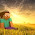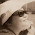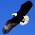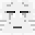## Wednesday, 27 February 2013

### Algebra... Which is larger? (I)

Which is larger in magnitude?
5 + n or  5n

This question is compulsory.

1.It depends. If n is 1, then 5+n(6) is more than 5n(5)
But if it is more than 1, 5n is more than 5+n.

1.Are you able to tell the number that determines which is larger?

2.5n. 5+n is addition, however 5n is 5 multiplied by n. Therefore, 5n is bigger

1.Is this always the case?

3.The answer is 5n.5+n is just n added to 5 whereas 5n is 5 multiplied to n.So 5n is bigger.

1.Are there exceptional cases?

4.5n. take n = 5.

therefore , 5n = 5 x 5 = 25
5 + n = 5 + 5 = 10

thus 5n is larger in magnitude

1.What happens when n = 0?

5.5n is bigger.
5+n is just adding the number5 and n.
5n is multiplying 5 with n.

1.What happens to 5n if n is a negative number?

6.5n. As 5n is 5*n, which is multiplication while 5+n is only addition

1.Test this out with a range of numbers!

7.it depends as if n is a negative no. then 5n will be smaller but if n is a positive no. then it will be bigger

1.The claim is, as long as n is positive, 5n is always bigger.
Test this out with positive proper fractions.

8.This comment has been removed by the author.

9.It depends. If n is 1 or if it is a negative number 5n will be smaller than 5+n. However if n is more than 1(not including), 5n will be bigger than 5+n

1.Is n = 1 the value that determines when which one is larger?

10.5n is larger. (If n is a positive integer) as compared to 5+n.

1.Ok, an assumption made is, n is an integer.
What if it's a set of real numbers? Does the claim still stand?

2.The things written on the board related to this qn.
If n=1, 5+n>5n
If n=2, 5+n<5n
If 5+n=5n, move n over to the 5n side to become -n. Thus, the equation becomes 5=5n-n=4n, n=5/4=1.25

11.If n is negative then 5n would be smaller (5 x -3=-15 is smaller than 5 + -3=2) ,
however if n is positive then 5n is bigger.
However if n were a decimal then it would be smaller. (0.2 x 5=1, 5+0.2=5.2)

1.Good observation.
So, what is the value that determines the change in 'status'?

12.5n is larger than 5+n because 5n is 5*n and 5 + n cannot be larger than 5 times of n

i.e. Take n = 5
5n = 5*5 = 25
5+n = 5+5 = 15
25 is therefore larger than 15.

1.I forgot to mention if n is a positive integer.

If n is a negative integer,

n=-5
5n = 5*-5 = -25
5 + n = 5 + -5 = 0
And therefore 0 is larger than 25 which means 5+n is the larger number now.

2.Good explanation.
This makes an assumption that the number is an integer.
What happens if n is a real number.
Does the same apply?

13.5+n is smaller than 5n as:
5+n= 5 PLUS n
5n= 5 TIMES n
Eg.: If n is 2 (this does not apply to 1 or numbers below it), it will become 5+2=7 and 5*2=10.

1.Elaborate your point further with illustrations

14.5n is larger than 5+n in magnitude because:
(Take n as 5)
5 + n = 5 + 5
= 10
5n = 5 x 5
= 25

5n is larger than 5+n in magnitude.

1.Is this true for all values of n?

15.5n is larger in magnitude

Example: n = 8
5+n = 5+8=13

5n = 5x8 = 40

Hence 5n is larger in magnitude

1.Try a wider range of n values

16.If n = 3
5n = 5*n
=5 *3
=15
5 + n = 5 + 3
=8
so 5n is greater than 5 +n

1.What if n is a negative number?
Does your claim still stand?

2.so if n = -3
5n = -15
5+n=5+(-3)
= 2
so 2 is greater than -15
so my claim still stands

17.IF we assume that n = 2, then
5n = 5*2
= 10
5 + n = 5 + 3
=15

1.What if we choose n to be a negative number?

18.so, 5 n is greater than 5 + n

19.5n is greater than 5 + n as you are multiplying 5 by n in 5n and you are adding 5 and n in 5+n.

1.Is this the case for all values of n? Test it out!

20.5n is larger because 5n is multiplied but 5+n is merely an addition. For example,
4+4=8 whereas 4x4=16. 16 is a bigger number than 8. Thus, 5n is a larger number.

21.If n>2, 5+n is bigger. However if n<1, 5n is bigger.

22.If n>2, 5+n will be bigger. However if n<1, 5n will be bigger.